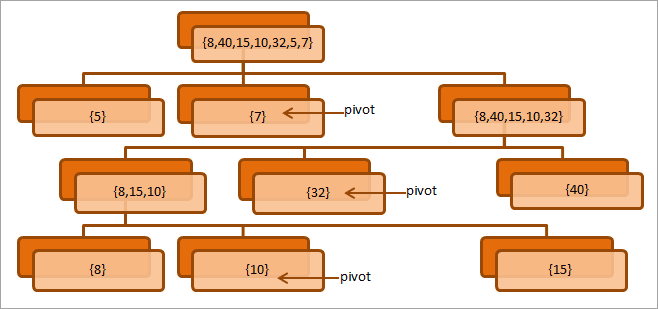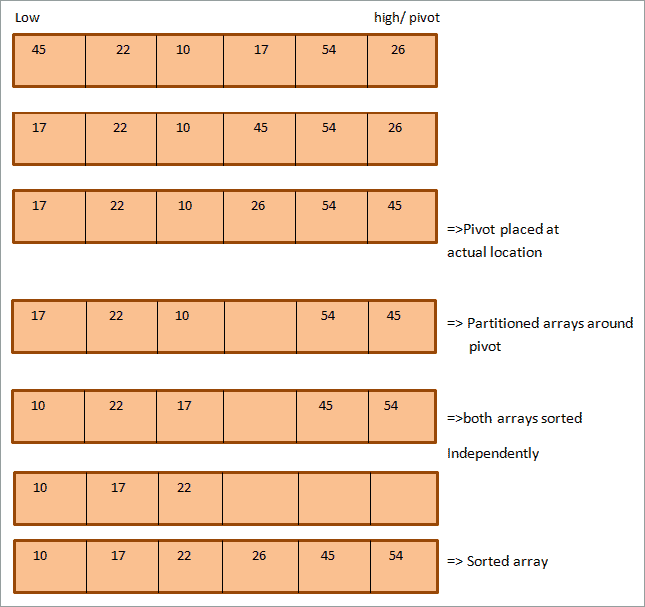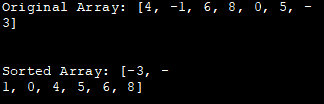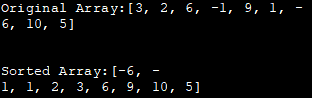# QuickSort In Java – Algorithm, Illustration & Implementation

This Tutorial Explains the Quicksort Algorithm in Java, its illustrations, QuickSort Implementation in Java with the help of Code Examples:

Quicksort sorting technique is widely used in software applications. Quicksort uses a divide-and-conquer strategy like merge sort.

In the quicksort algorithm, a special element called “pivot” is first selected and the array or list in question is partitioned into two subsets. The partitioned subsets may or may not be equal in size.

The partitions are such that all the elements less than the pivot element are towards the left of the pivot and the elements greater than the pivot is at the right of the pivot. The Quicksort routine recursively sorts the two sub-lists. Quicksort works efficiently and also faster even for larger arrays or lists.

• Quicksort Partition Java
• Quicksort Algorithm Java
• Pseudocode For Quick Sort
• Illustration
• Quicksort Implementation In Java
• Recursive Quicksort
• Iterative Quicksort
• Frequently Asked Questions
• Conclusion
• Recommended Reading

### Quicksort Partition Java

Partitioning is the key process of the Quicksort technique. So what is partitioning?

Given an array A, we choose a value x called pivot such that all the elements lesser than x are before x, and all the elements greater than x are after x.

A pivot value can be any of the following:

• The first element in the array
• The last element in the array
• The mid element in the array
• Any random element in the array

This pivot value is then placed at its proper position in the array by partitioning the array. Thus the output of the ‘partitioning’ process is the pivot value at its proper position and the elements less than pivot on the left and elements greater than a pivot at the right.

Consider the following diagram that explains the partitioning process.The above diagram shows the process of partitioning array by repeatedly selecting the last element in the array as a pivot. At each level, note that we partition the array into two sub-arrays by placing pivot at its correct position.

Next, we list the algorithm and pseudo-code for quicksort technique that also includes partition routine.

### Quicksort Algorithm Java

The general algorithm for quicksort is given below.

 `quicksort(Arr, low, high)``begin``    ``Declare array Arr[N] to be sorted``    ``low = 1st element; high = last element; pivot ``    ``if``(low < high)``    ``begin``        ``pivot = partition (Arr,low,high);``        ``quicksort(Arr,low,pivot-``1``)``        ``quicksort(Arr,pivot+``1``,high)     ``    ``end``end`

Given below is the pseudo-code for the quicksort technique.

### Pseudocode For Quick Sort

Following is the pseudo-code for a quick sort sorting technique. Note that we have provided the pseudo-code for quicksort and partitioning routine.

 `//pseudocode for quick sort main algorithm``procedure quickSort(arr[], low, high)``    ``arr = list to be sorted``    ``low – first element of the array``    ``high – last element of array``begin``    ``if` `(low < high)``    ``{``       ``// pivot – pivot element around which array will be partitioned ``        ``pivot = partition(arr, low, high);``        ``quickSort(arr, low, pivot - ``1``);  ``// call quicksort recursively to sort sub array before pivot``        ``quickSort(arr, pivot + ``1``, high); ``// call quicksort recursively to sort sub array after pivot``    ``}``end procedure``//partition routine selects and places the pivot element into its proper position that will partition the array. ``//Here, the pivot selected is the last element of the array``procedure partition (arr[], low, high)``begin``    ``// pivot (Element to be placed at right position)``    ``pivot = arr[high];  ``     ``i = (low - ``1``)  ``// Index of smaller element``    ``for` `j = low to high``    ``{``        ``if` `(arr[j] <= pivot)``        ``{``            ``i++;    ``// increment index of smaller element``            ``swap arr[i] and arr[j]``        ``}``    ``}``    ``swap arr[i + ``1``] and arr[high])``    ``return` `(i + ``1``)``end procedure`

### Illustration

Let’s see the illustration of the quicksort algorithm. Take the following array as an example. Here we have selected the last element as pivot.

As shown, the first element is labeled low and the last element is high.As evident in the above illustration, there are two pointers, high and low that respectively point to the last and first elements of the array. Both these pointers are moved as the quicksort progresses.

When the element pointed by the low pointer becomes greater than the pivot element and element pointed by the high pointer is lesser than the pivot element, we exchange the elements pointed by the low and high pointer, and each pointer advances by 1 position.

The above steps are carried out until both the pointers cross each other in the array. Once they cross, the pivot element gets its proper position in the array. At this point, the array is partitioned and now we can sort each sub-array independently by recursively applying a quick sort algorithm to each of the sub-array.

### Quicksort Implementation In Java

QuickSort technique can be implemented in Java using either recursion or iteration. In this section, we will see both of these techniques.

#### Recursive Quicksort

We know that the basic technique of quicksort illustrated above uses recursion for sorting the array. In the recursive quicksort after partitioning the array, the quicksort routine is called recursively to sort the sub-arrays.

The below Implementation demonstrates the quicksort technique using recursion.

 `import` `java.util.*;``class` `QuickSort { ``    ``//selects last element as pivot, pi using which array is partitioned. ``    ``int` `partition(``int` `intArray[], ``int` `low, ``int` `high) { ``        ``int` `pi = intArray[high];  ``        ``int` `i = (low-``1``); ``// smaller element index   ``        ``for` `(``int` `j=low; jpartitioning index and return pi``            ``int` `pi = partition(intArray, low, high); ``  ``            ``// sort each partition recursively ``            ``quick_sort(intArray, low, pi-``1``); ``            ``quick_sort(intArray, pi+``1``, high); ``        ``} ``    ``} ``}``class` `Main{``    ``public` `static` `void` `main(String args[]) {``        ``//initialize a numeric array, intArray``        ``int` `intArray[] = {``4``,-``1``,``6``,``8``,``0``,``5``,-``3``}; ``        ``int` `n = intArray.length; ``        ``//print the original array``        ``System.out.println(``"Original Array: "` `+ Arrays.toString(intArray));``        ``//call quick_sort routine using QuickSort object``        ``QuickSort obj = ``new` `QuickSort(); ``        ``obj.quick_sort(intArray, ``0``, n-``1``); ``        ``//print the sorted array``        ``System.out.println(``"\nSorted Array: "` `+ Arrays.toString(intArray)); ``    ``} ``}`

Output:

Original Array: [4, -1, 6, 8, 0, 5, -3]
Sorted Array: [-3, -1, 0, 4, 5, 6, 8]#### Iterative Quicksort

In iterative quicksort, we use the auxiliary stack to place intermediate parameters instead of using recursion and sort partitions.

The following Java program implements iterative quicksort.

 `import` `java.util.*; ``  ``class` `Main { ``    ``//partitions the array around pivot=> last element``    ``static` `int` `partition(``int` `numArray[], ``int` `low, ``int` `high)   { ``        ``int` `pivot = numArray[high]; ``        ``// smaller element index ``        ``int` `i = (low - ``1``); ``        ``for` `(``int` `j = low; j <= high - ``1``; j++) { ``            ``// check if current element is less than or equal to pivot``            ``if` `(numArray[j] <= pivot) {``                ``i++; ``                ``// swap the elements``                ``int` `temp = numArray[i]; ``                ``numArray[i] = numArray[j]; ``                ``numArray[j] = temp; ``            ``} ``        ``} ``        ``// swap numArray[i+1] and numArray[high] (or pivot) ``        ``int` `temp = numArray[i + ``1``]; ``        ``numArray[i + ``1``] = numArray[high]; ``        ``numArray[high] = temp; ``        ``return` `i + ``1``; ``    ``} ``  ``    ``//sort the array using quickSort``    ``static` `void` `quickSort(``int` `numArray[], ``int` `low, ``int` `high) ``    ``{ ``        ``//auxillary stack``        ``int``[] intStack = ``new` `int``[high - low + ``1``]; ``  ``        ``// top of stack initialized to -1``        ``int` `top = -``1``; ``  ``        ``// push initial values of low and high to stack ``        ``intStack[++top] = low; ``        ``intStack[++top] = high; ``  ``        ``// Keep popping from stack while is not empty ``        ``while` `(top >= ``0``) { ``            ``// Pop h and l ``            ``high = intStack[top--]; ``            ``low = intStack[top--]; ``  ``            ``// Set pivot element at its correct position ``            ``// in sorted array ``            ``int` `pivot = partition(numArray, low, high); ``  ``            ``// If there are elements on left side of pivot, ``            ``// then push left side to stack ``            ``if` `(pivot - ``1` `> low) { ``                ``intStack[++top] = low; ``                ``intStack[++top] = pivot - ``1``; ``            ``} ``  ``            ``// If there are elements on right side of pivot, ``            ``// then push right side to stack ``            ``if` `(pivot + ``1` `< high) { ``                ``intStack[++top] = pivot + ``1``; ``                ``intStack[++top] = high; ``            ``} ``        ``} ``    ``}``public` `static` `void` `main(String args[]) ``    ``{ ``        ``//define array to be sorted``        ``int` `numArray[] = { ``3``,``2``,``6``,-``1``,``9``,``1``,-``6``,``10``,``5` `}; ``        ``int` `n = ``8``; ``        ``System.out.println(``"Original Array:"` `+ Arrays.toString(numArray)); ``        ``// call quickSort routine to sort the array ``        ``quickSort(numArray, ``0``, n - ``1``); ``        ``//print the sorted array``        ``System.out.println(``"\nSorted Array:"` `+ Arrays.toString(numArray)); ``    ``} ``}`

Output:

Original Array:[3, 2, 6, -1, 9, 1, -6, 10, 5]
Sorted Array:[-6, -1, 1, 2, 3, 6, 9, 10, 5]### Frequently Asked Questions

Q #1) How does a Quicksort work?

Answer: Quicksort uses a divide and conquers strategy. Quicksort first partitions an array around a pivot element selected and generates sub-arrays that are sorted recursively.

Q #2) What is the time complexity of Quicksort?

Answer: The time complexity of quicksort on an average is O (nlogn). In the worst case, it is O (n^2) the same as the selection sort.

Q #3) Where is Quicksort used?

Answer: Quicksort is mostly used in recursive applications. Quicksort is the part of C-library. Also, almost the programming languages that use built-in sorting implement quicksort.

Q #4) What is the advantage of Quicksort?

Answer:

• Quicksort is an efficient algorithm and can easily sort even a huge list of elements.
• It is in-place sort and hence does not need extra space or memory.
• It is widely used and provides an efficient way to sort data sets of any length.

Q #5) Why is Quicksort better than the merge sort?

Answer: The main reason for which the quicksort is better than the merge sort is that quicksort is in-place sorting method and does not require additional memory space. Merge sort requires additional memory for intermediate sorting.

### Conclusion

Quicksort is considered as the best arranging calculation fundamentally as a result of its proficiency to sort even a tremendous informational collection in O (nlogn) time.

Quicksort is also an in-place sort and doesn’t require additional memory space. In this tutorial, we have seen the recursive and iterative implementation of quicksort.